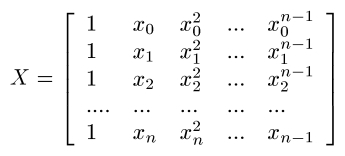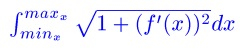## Wednesday, January 16, 2013

### Length of a Polynomial Segment - Part 2 (TI-84+/Casio); From Coffee Klatch in San DimasToday I am coming to you from Coffee Klatch in San Dimas, CA. This is one of my most favorite places to hang out, and have great coffee and food.

Today's blog is the second part of the polynomial segment blog. Here is a link to Part 1.

To Recall:

A polynomial segment is a set of points that is connected by a polynomial.

To find a perfectly fit polynomial to fit n points:

1. Let X be the Vandermonde matrix of order (n-1) × (n-1) for the following:2. Let y be the vector of y values.
3. The coefficient matrix is calculated as (X^T X)^-1 (X^T y). The result is a vector of coefficients of x^k where k = 0 to n-1.

On most graphing (and some scientific calculators), you can accomplish this task in two ways:

1. Use matrix operations. This is good for any size polynomial.
2. Take advantage of the curve fitting functions LinReg (to fit 2 points), QuadReg (to fit 3 points), CubicReg (to fit 4 points), and QuartReg (to fit 5 points). *Regression models and names vary for each calculator.

Once the polynomial, f(x) is found, find the arc length is found by:The limits of the integral are the minimum and maximum x values, respectively.

Here are two programs that accomplish the task of finding a polynomial fit for 3, 4, or 5 points for the TI-84+ (and its family), and the Casio Prizm (and its fx-9xxx family).

TI-84+

POLYARC
1/12/2013
Fit a polynomial to a set of three, four, or five points.
Find the arc length of the polynomial from the end points.

Example 1: (0,0), (4,8), (8,6)
Results: y = -0.3125x^2 + 3.25
Length = 14.23920

Example 2: (0,3), (2,-1), (4,2), (6,0)
Results: y = -0.25x^3 + 2.375x^2 - 5.75x + 3
Length = 12.46834

Example 3: (0,0), (1,1), (2,0), (3,-1), (4,0.5)
Results: y ≈ -0.02083333x^4 + 0.20833333x^3 - 1.77083333x^2 + 2.54166666x
Length = 6.36142413

Program POLYARC (327 bytes)
Input "NO OF PTS(3-5)", N
If N<3 or N>5
1/0
N → dim(L1)
N → dim(L2)
For(K,1,N)
Disp K
Input "X=", X
Input "Y=", Y
X → L1(K)
Y → L2(K)
End
If N=3
Then
fnInt(√(1+(2aX+b)²),X,min(L1),max(L1)) → L
Pause {a, b, c}
End
If N=4
Then
CubicReg L1, L2
Pause {a, b, c, d}
fnInt(√(1+(3aX² + 2bX + c)²), X, min(L1), max(L1)) → L
End
If N=5
Then
QuartReg L1, L2
Pause {a, b, c, d, e}
fnInt(√(1 + (4aX³ + 3bX² + 2cX + d)² , X, min(L1), max(L2)) → L
End
Disp "LENGTH="
Pause L

Where to find:
a, b, c, d, e: VARS, 5 (Statistics), right, right, [2, 3, 4, 5, and 6 respectively]
You need the lower case a, b, c, d, and e!

Note: No "bending backwards" is allowed

Casio Prizm

POLYARC
1/12/2013
Fit a polynomial to a set of three or four points.
Find the arc length of the polynomial from the end points.

Example 1: (0,0), (4,8), (8,6)
Results: y = -0.3125x^2 + 3.25
Length = 14.23920

Example 2: (0,3), (2,-1), (4,2), (6,0)
Results: y = -0.25x^3 + 2.375x^2 - 5.75x + 3
Length = 12.46834

Example 3: (0,0), (1,1), (2,0), (3,-1), (4,0.5)
Results: y ≈ -0.02083333x^4 + 0.20833333x^3 - 1.77083333x^2 + 2.54166666x
Length = 6.36142413

Program POLYARC (352 bytes):
"NO. OF POINTS(3,4,5)"? → N
N<3 Or N>5 ⇒ 1 ÷ 0
N → Dim List 1
N → Dim List 2
For 1 → K To N
K ◢
"X"? → List 1[K]
"Y"? → List 2[K]
Next
If N = 3
Then QuadReg List 1, List 2
∫( √(1 + (2aX + b)² ), Min(List 1), Max(List 1)) → L
{a, b, c} ◢
EndIf
If N=4
Then CubicReg List 1, List 2
∫( √(1 + (3aX ² + 2bX + c)² ), Min(List 1), Max(List 1)) → L
{a, b, c, d} ◢
EndIf
If N=5
Then QuartReg List 1, List 2
∫ ( √(1 + (4aX^3 + 3bX ² + 2cX + d)² ), Min(List 1), Max(List 2)) → L
{a, b, c, d, e} ◢
EndIf
"LENGTH="
L

How to Access:
a, b, c, d, and e: VARS, STAT (F3), GRAPH (F3), F1 (F2, F3, F4, and F5 respectively)

Note: No "bending backwards" allowed

Enjoy the day and I'll talk to you soon!

Eddie

This blog is property of Edward Shore. 2013

### Casio fx-CG50: Sparse Matrix Builder

Casio fx-CG50: Sparse Matrix Builder Introduction The programs can create a sparse matrix, a matrix where most of the entries have zero valu...